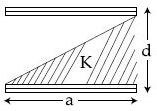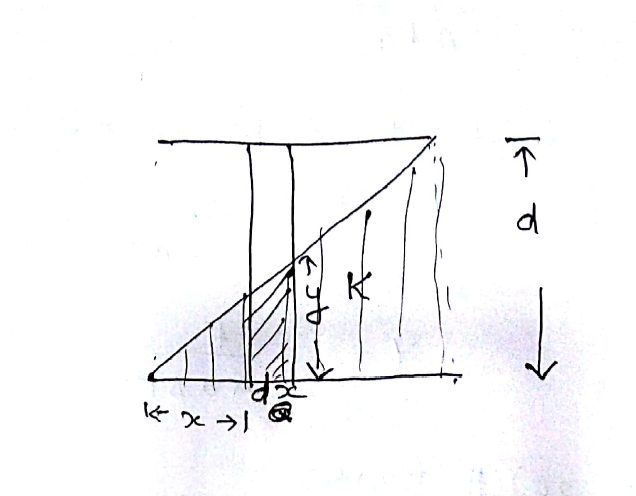Q

# Answer please! A parallel plate capacitor is made of two square plates of side 'a' , separated by a distance d (d>>a). The lower triangular portion is filled with a dielectric of dielectric constant K, as shown in the figure. Capacitance of

A parallel plate capacitor is made of two square plates of side 'a' , separated by a distance d (d>>a). The lower triangular portion is filled with a dielectric of dielectric constant K, as shown in the figure. Capacitance of this capacitor is :• Option 1)

• Option 2)

• Option 3)

• Option 4)

Views

If K filled between the plates -

- whereinOption 1)

Option 2)

Option 3)

Option 4)

Exams
Articles
Questions